# Use a current mirror to control a power supply

## NXP BCV61

Giovanni Romeo

EDN

Controlling a power supply is a necessary task in many applications, such as in battery chargers, solar power controllers, and so on. Industry provides a rich number of off-the-shelf integrated power supplies that, unfortunately, do not offer a simple way to control the output. Usually a power supply can be schematized as a power operational amplifier with the non-inverting input connected to a reference voltage (in the green rectangle in Figure 1).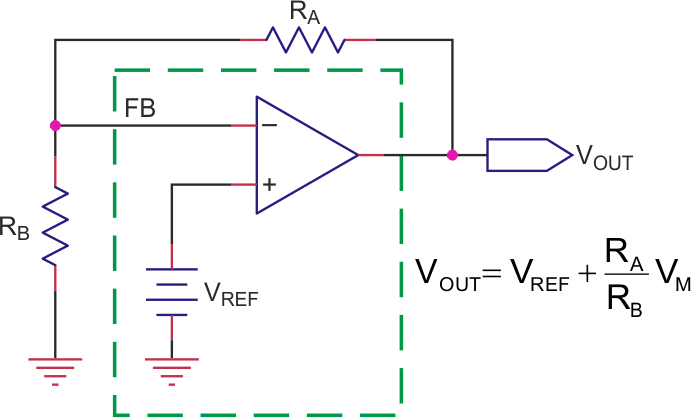Figure 1. This is a feedback-stabilized power supply scheme.

Typically, in power supply ICs (i.e. TI’s Simple Switcher) the only access you have to change VOUT is the inverting pin that controls the feedback (FB in Figure 1). A very easy method to control FB is to replace RB with a controllable current source, and the simplest and cheapest way to do that is using a current mirror (Figure 2).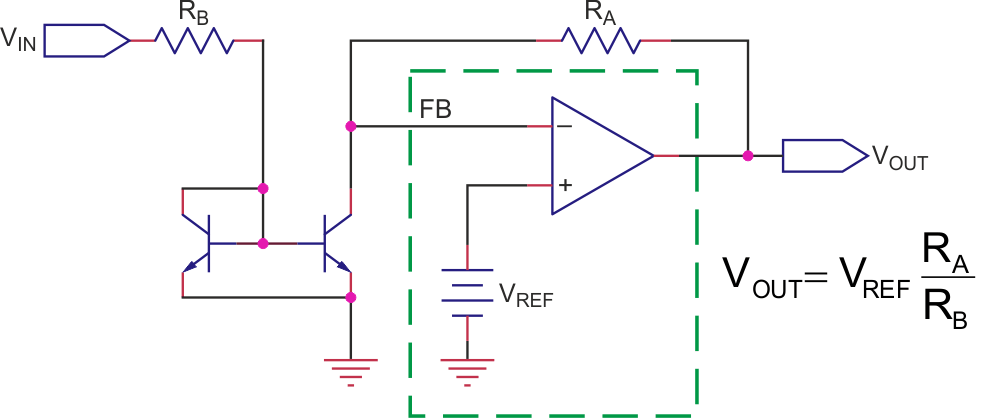Figure 2. This voltage-controlled power supply uses a current mirror.

The precision you get from this design is tied to the precision of the current mirror you’ll use. If you decide for the Widlar basic two-transistor design, it’s important to rely on matched pairs built on purpose, like the BCV61; it’s easy to use such components in the better performing Wilson 4-transistors current mirror. The current mirror starts working only when VIN exceeds the VBE(on) of the mirror transistors, so there is a non-linearity at the beginning. All this is not very constraining if the suggested design is part of a loop where errors are compensated by the feedback magic.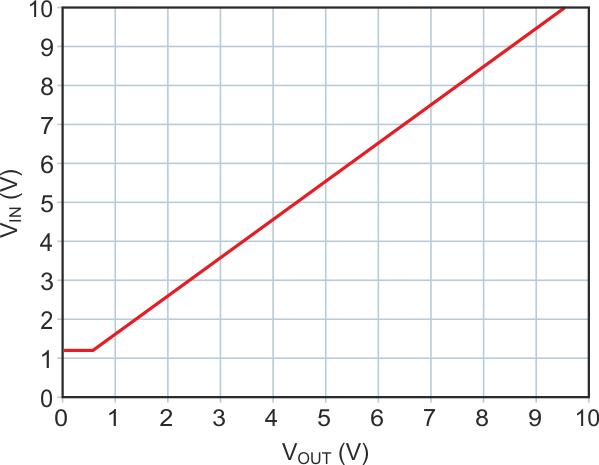Figure 3. This diagram shows the PSpice simulated comportment of the circuit in Figure 2.

The diagram in Figure 3 shows the PSpice simulated comportment of the Figure 2 circuit with VREF = 1.2 V when VIN spans from 0 to 10 V.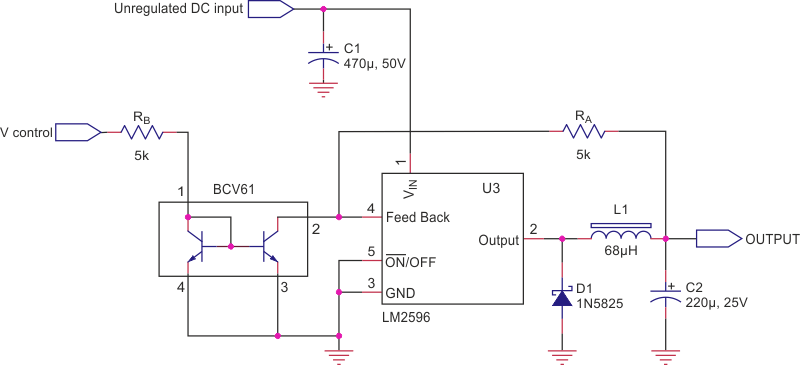Figure 4. Here is a ‘designed-to-work’ application of the principle of Figure 2.

A straightforward implementation of the principle depicted in Figure 2 appears in Figure 4. Here, the well-known LM2596 is controlled by the off-the-shelf current mirror BCV61.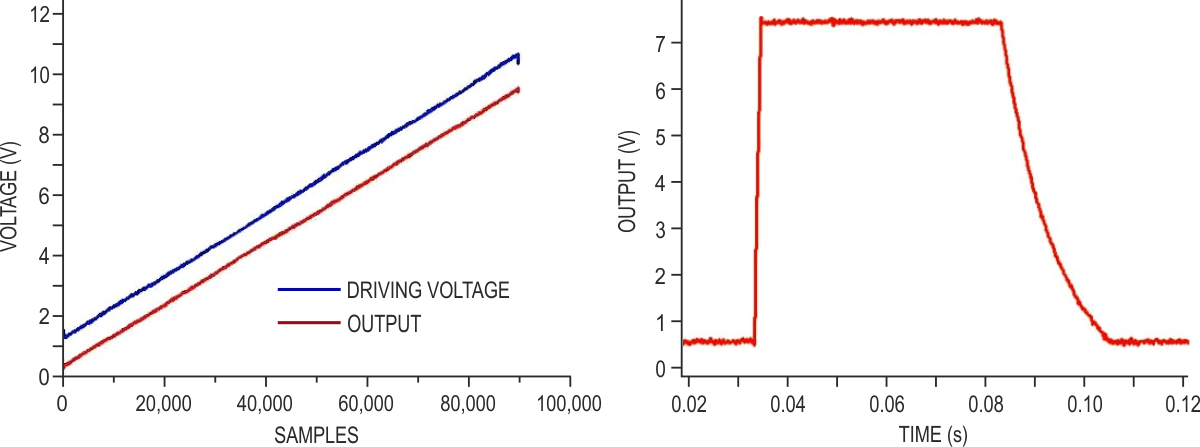Figure 5. These test results for the circuit of Figure 4 show the driving voltage (blue track), and the output (red track on left). On the right, the response to a square wave shows a 1 ms rising time and a slow falling time tied to the output capacitor and the load resistor.

The prototype of Figure 4 has been tested for linearity by connecting the unregulated DC input to a 22 V power supply, V control to a sawtooth generator spanning 0-10 V @ 5 Hz, and sampling the output (loaded with a 50 Ω resistor) with a scope. A pulse generator (0-8 V, 0.5 s) has been used to check the time response.

The results are shown in Figure 5. The circuit offers a good linearity (left) and a quite fast transient response for the rising time (roughly 1 ms to reach the settling point). The falling time is tied to the output capacitor (220 µF) and to the load (50 Ω during the test).

EDN